# 15.10 - Optimizer Join Plans - Teradata Database

## Teradata Database SQL Request and Transaction Processing

prodname
vrm_release
15.10
category
Programming Reference
User Guide
featnum
B035-1142-151K

Selecting the optimum method to join relations is as critical to the performance of a query as the selection of table access methods. Selecting an optimal join method, or mode, is a separate issue from that of determining the optimal join order for a query. Join order optimization is described in “Evaluating Join Orders” on page 376.

There are many methods for joining relations, and the method ultimately chosen to make a join depends on multiple factors, including the comparison on which the join is made (vanilla equijoin or Θ join, where Θ is any valid non‑equality condition) A Q join is not necessarily made on an inequality condition, but the term generally implies an inequality.), the estimated cardinality of the relations being joined, the configuration of the system on which the join is to be made, and so on.

This section of the chapter describes join processing at several levels including the important topic of join methods.

Join planning involves the following core components:

• Selecting a join method.
• There are often several possible methods that can be used to make the same join. For example, it is usually, but not always, less expensive to use a merge join rather than a product join. The choice of join method often has a major effect on the overall cost of processing a query.

• Determining an optimal join geography.
• Different methods of relocating rows to be joined can have very different costs. For example, depending on the size of the relations in a join operation, it might be less costly to duplicate one of the relations rather than redistributing it.

• Determining an optimal join order.
• Only two relations can be joined at a time. The sequence in which relation pairs are joined can have a powerful impact on join cost. In this context, a table could be joined with a spool rather than another table. The term table is used in the most generic sense of the word, and the logical term relation is often used as a substitute for any table, view, or spool.

• Determining the optimal spool format.
• Teradata can select regular format or in-memory format, depending on the cost. The cost model uses various parameters, including the production of an in-memory spool. In some cases the optimizer converts a regular table to an in-memory spool format table.

The following table outlines some of the terminology introduced by join planning:

 Term Type Definition Example Bind An equality constraint between two columns of different relations. Normally used to determine whether there are conditions on an index. `(t1.a = t2.a)` Cross A predicate with expressions on different relations. A cross term can be generated for a condition of the form `column_name=expression`, which is referred to as a half cross term. The Optimizer can use a half cross term as a constraint if it is specified on an index. The form `conversion(column)=expression` can be used for the same purpose if `conversion(column)` and `column` are hashed the same. `(t1.a = t2.a + 1)` Exists A predicate that specifies an EXISTS condition. Explicit A predicate defined on a constant. Normally used to determine whether there are conditions on an index. `(t1.a = 1)` Minus A predicate that specifies a MINUS condition. Miscellaneous Literally a group of terms that do not belong to any of the other categories. The set of miscellaneous terms includes the following: Inequality terms. Equality terms on either the same set of relations or on non‑disjoint sets of relations. ANDed terms. ORed terms. Other terms (for example, terms expressed over more than 2 relations). A miscellaneous term can be generated for a `conversion(column) = constant` condition. If `conversion(column)` and `(column)` hash the same, then the miscellaneous term points to an explicit term. For example, if the operation undertaken by the conversion is a simple renaming of the column. In this case, the miscellaneous term is used to determine whether a constraint is specified on an index column. Outer join An outer join term. Geospatial column terms are not permitted for outer join conditions.

The first thing the Optimizer looks for when planning a join is connecting conditions, which are predicates that connect an outer query and a subquery. The following are all examples of connecting conditions:

`     (t1.x, t1.y) IN (SELECT t2.a, t2.b FROM t2)`
`     → (t1.x IN spool1.a AND (t1.y IN spool1.b)`
` `
`     (t1.x, t1.y) IN (SELECT t2.a, constant FROM t2)`
`     → (t1.x IN spool1.a) AND (t1.y=spool1.constant)`
` `
`     (t1.x, constant) NOT IN (SELECT t2.a, t2b FROM t2)`
`     → (t1.x NOT IN spool1.a) AND (constant NOT IN spool1.b)`

The following information provides a general overview of how the Optimizer analyzes conditions to determine the connections between relations in a join operation:

• There is a direct connection between two relations if either of the following conditions is found:
• An ANDed bind, miscellaneous, cross, outer join, or minus term that satisfies the dependent info between the two relations.
• A spool of an uncorrelated subquery EXIST condition connects with any outer relation.
• Geospatial column terms are not permitted for outer join conditions.

• An ANDed miscellaneous or explicit term on a single relation is pushed to the relation.
• A term on no relation is pushed to a relation.
• An ORed term that references some subqueries and a single relation is associated with that relation as a complex term.
• All relations that are referenced in an ORed term that specifies subqueries and more than one relation are put into complex set.
• All relations that are specified in some join condition are marked as connected.
• Assume selection and projection are done if a relation is spooled before join, for example:
• `     SELECT t1.x1 `
`     FROM t1, t2 `
`     WHERE y1=1 `
`     AND   x1= x2;`
• Find the following information about all relations in the set of input relations:
• Its row size after applying projection.
• Its cardinality after applying selection conditions.
• The cost to read it based on the previously determined row size and cardinality.
• Its output row cost.
• The maximum cardinality is used to estimate the cost of a nested join.
• The poorest cardinality estimate is displayed in the EXPLAIN text for the query.
• Its primary index if it has one.
• A pointer to table descriptor of useful indexes.
• The set of input relations for the join.
• A flag to indicate whether the rows are sorted by the primary index
• The set of connected relations such as the following.
• `     SELECT t1.x1 `
`     FROM t1, t2 `
`     WHERE y1=1 `
`     AND   x1= x2;`
• Find the following information about all base table relations in the set of input relations:
• The relation row size.
• The relation cardinality.
• The selection condition list.
• The projection list.
• The best possible access paths (using calls to access planning functions).
• Find the following information about all spool relations in the set of input relations:
• The spool cardinality
• The selection condition list.
• Its assignment list.
• Its spool number.
• Depending on the indexes defined for the tables involved and whether statistics are available for the indexes, the Optimizer processes a join using one of the following basic join methods.

• Product join
• Hash join
• In-memory hash join
• Merge join
• Nested join (local and remote)
• Exclusion join (merge and product)
• Inclusion join (merge and product)
• RowID join
• Self-join
• Correlated join
• Minus all join
• See “Join Strategies and Methods” on page 392 and the pages following for more information about each of these join methods.

Excluding self-joins, as many as 128 tables or single‑table views can be joined per query block. The maximum number of tables and single‑table views that can be joined per query block is determined by the value of the MaxJoinTables cost profile option parameter, which ranges from a minimum of 16 to a maximum of 128, and the MaxJoinTables performance field of the DBS Control record, which ranges from a minimum of 64 to a maximum of 128. See Utilities for details.

Loosely defined, a query block is a unit for which the Optimizer attempts to build a join plan. The following list notes a few of the more frequently occurring query blocks:

• Uncorrelated subqueries
• Derived tables
• Complicated views
• Portions of UNION and INTERSECT operations
• Each reference to a relation, including those using correlation names, counts against the limit of 128 tables. This limit includes implicit joins such as the join of a hash or single-table join index to a base table to process a partial cover.

For example, consider a query with a single uncorrelated subquery. The subquery is limited to 128 tables and the outer query is limited to 127 tables, the 128th table for the outer query being the spooled result of the inner query that must be joined with it.

If the uncorrelated subquery were an outer query to an additional uncorrelated subquery, then the deepest subquery would be limited to referencing 128 tables, its outer query limited to 127 (127 plus the result of its inner query), and the parent outer query to 127 plus the result of its inner query.

In summary, while the number of tables, including intermediate spool relations, that can be joined is limited to 128 per query block, the cumulative number of tables referenced in the course of optimizing the query can be considerably greater than 128.

There is no way to determine a priori how many query blocks will be created and processed by the Optimizer in the course of producing a join plan, but the factors listed here are all candidates to evaluate if your queries terminate because they exceed the 128 table join limit.

Always join tables and views on columns that are defined on the same domain.

If the join columns are not defined on the same domain, then the system must convert their values to a common data type before it can process the join (see SQL Functions, Operators, Expressions, and Predicates for information about implicit data type conversions). The conversion process is resource‑intensive and thus a performance burden.

Distinct user‑defined data types (UDTs) are a good method of defining domains that also bring strong typing into the picture, eliminating the possibility of implicit type conversions unless the UDT designer explicitly codes them.

This positive is balanced by the negative that you cannot define constraints on UDT columns. Because of this limiting factor, whether distinct UDTs are a good way for you to define your domains or not is a matter of balancing several factors and determining which method, if either, best suits domain definitions in your development environment.

See SQL External Routine Programming and SQL Data Definition Language for information about UDTs. See Database Design for information about using UDTs to define domains.

If you plan to join more than 32 relations in a request, use the DBS Control Utility (see Utilities) to increase the value for the DBS Control Record MaxParseTreeSegs performance field to something greater than the default value of 2,000 parse tree memory segments allocated to Parser memory for code generation.

 THE minimum value for MaxParseTreeSegs is … THE default value for MaxParseTreeSegs is … AND the maximum value for MaxParseTreeSegs is … 12 2,000 12,000

The MaxParseTreeSegs field defines the maximum number of 64 KB parse tree memory segments the Parser allocates when parsing an SQL request. Teradata Database allocates the parse tree segments dynamically as they are needed, so this field does not unnecessarily preallocate memory that might not be used.

Because that the Optimizer is cost‑based, it evaluates the relative costs of the available join methods (see “Join Strategies and Methods” on page 392) to determine the least expensive method of joining two relations.

Product joins (see “Product Join” on page 398) tend to be the most costly join method, and the system uses them only when it produces a better plan than a merge join (see “Merge Join” on page 405). For equality joins only, a hash join (see “Hash Join” on page 434) can be the least costly join method, particularly if the smaller relation of the join pair is small enough that it can all fit into memory. In this case, a special hash join called a dynamic hash join can be used (see “Dynamic Hash Joins” on page 443).

As an example, consider an equality join. In this case, the smaller relation is duplicated on all AMPs and then every row in the smaller relation is compared with every row in the larger relation. The total number of comparisons to do the join is the product of the number of rows in the smaller relation and the number of rows in the larger relation.

An optimal solution for reducing the number of comparisons made by a product join is to assign the smaller relation rows to a hash array and then use a hash join instead of a product join. In this case, each row in the right relation does a simple table look up in the hash array to see if an entry exists or not.

 IF there is … THEN the system … no match in the hash array discards the row and makes no further comparisons. a match in the hash array continues processing to determine if the remaining join conditions match.

No other entries in the hash array need to be checked.

For an equality product join, if the estimated cardinality of the small relation is 5 rows and the actual cardinality is 500 rows, then each row in the right relation would have to make 500 comparisons instead of the estimated 5 comparisons. This is a difference of two orders of magnitude.

For a hash join, each row in the right relation only needs to make one probe into the hash array. To make this join even more efficient, Teradata Database can compute the row‑hash code dynamically instead of taking an extra step to create a spool containing the row hash code based on the join columns. This optimization duplicates the left relation and generates the right relation hash codes dynamically (see “Dynamic Hash Joins” on page 443).

Replacing the equality product join with a dynamic hash join also allows for other optimizations. For example, suppose a system has more than 100 AMPs. The smaller relation in a join has 100 rows evenly distributed across the AMPs.

Consider the following three join options for these relations. The options are numbered for reference purposes, not for precedence:

1 The Optimizer can generate a join plan to redistribute the larger relation and do a product join with approximately one row per AMP.

The join can be on the primary index of the small relation, which means redistributing the small relation is unnecessary, or the join could be on a non‑primary index set of columns. In the latter case, the small relation will also have to be redistributed. After redistributing the larger relation, only one comparison per row, on average, is needed because there is only one row per AMP in the small relation.

2 The Optimizer can decide to duplicate the smaller relation and then do a product join with 100 rows so that every row in the large relation requires 100 comparisons.

The usefulness of this option depends on the size of the larger relation.

3 The Optimizer can decide to duplicate the smaller relation and then sort both the large and small relations followed by a merge join.

Option 1 is faster than Option 2. In Option 1, rows from the large relation are read from the disk and redistributed to another AMP, so there is a higher preparation cost, but a much lower join cost than Option 2.

Similarly, there is a higher preparation cost in Option 3, than Option 2 because the large relation needs to be sorted. However, by replacing the equality product join with a hash join, the smaller relation can be duplicated instead of redistributing the larger relation. The comparison costs are approximately the same because only one comparison per row is required in both cases. The larger relation only needs to be read once and it does not have to be sorted. In other words, this method provides the benefit of a lower preparation cost as well as a lower join cost by using a hash join.

Note that the ordering of joins is a separate process from the selection of methods to use for those joins. See “Evaluating Join Orders” on page 376 for information about how the Optimizer evaluates join orders.

Unique secondary indexes (USIs) and nonunique secondary indexes (NUSIs) are used in a join operation only if the join plan used by the Optimizer calls for a nested join or a rowID join.

If more than two relations are specified in a join, the Optimizer reduces that join to a series of binary joins and then attempts to determine the most cost effective join order.

For example, assume you submitted the following multitable request:

`     SELECT ...`
`     FROM A, B, C, D`
`     WHERE ...;`

The Optimizer generates join plans like the three diagrammed in the following graphic. Note that relational database management systems do not perform physical 3-way joins as the join plan at the extreme left of the diagram suggests by joining the AB spool with relations C and D. The 3-way join plan in the diagram is provided for conceptual purposes only. Because the Optimizer uses column statistics to choose the least costly join plan from the candidate set it generates, the plans it generates are extremely dependent on the accuracy of those numbers.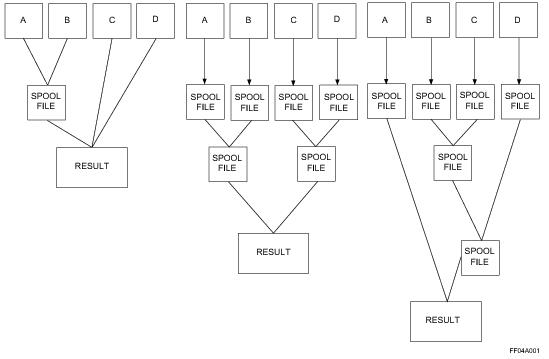Column projection and row selection are done prior to doing the join. If selection criteria are not supplied, then all rows and columns participate in the join process.

Obviously, it is always advantageous to reduce the number of rows and columns that must be duplicated or redistributed; however, column projections are done only when it is more efficient to do so. If a join can be done directly with the base table, column projection is not done before the join.

Query optimizers use trees to build and analyze optimal join orders. The join search tree types used most frequently by commercial relational database optimizers are the left-deep tree and the bushy tree (Ioannidis and Kang, 1991).

When a left-deep search tree is used to analyze possible join orders, the number of possibilities produced is relatively small, as characterized by the following equation, where n is the number of relations being joined.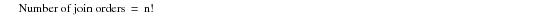For a 4‑relation join, the number of join orders using a left-deep search tree is only 4!, or 24. Left-deep join trees are used by many commercial relational systems, but there are other methods that can produce many more possible join sequences, making it more likely to find a better join plan.

The following graphic illustrates a left-deep join tree for a 4‑relation join: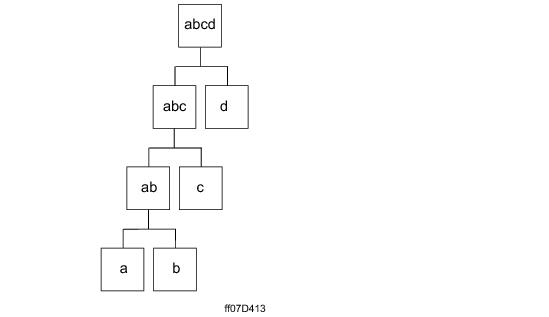Bushy trees are an optimal method for generating more join order combinations. At the same time, the number of combinations generated can be prohibitive (see “Possible Join Orders as a Function of the Number of Relations To Be Joined” on page 362), so the Optimizer uses several heuristics to prune the search space. For example, with the exception of star joins, bushy search trees are not considered for unconstrained joins.

Because the optimization of join orders is known to be NP‑complete (Ibaraki and Kameda, 1984), all query optimizers employ sets of heuristic devices to constrain the join space to a reasonable size. This is a necessity because the complexity of the join order space is on the order of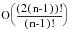(Seshadri et al., 1996).

where:

 Equation element … Represents the … O Landau complexity symbol of number theory and computation theory, meaning “order of.” n number of relations in the join.

Complexity can be further reduced to O(n3) by pursuing only bushy plans that exclude Cartesian products, to O(3n) for bushy plans inclusive of Cartesian products, and to O(n2n) for left‑deep searches (Ono and Lohman, 1990).

The following equation calculates the total number of join orders (before pruning) for n relations based on a bushy join tree search:The following term in this equation represents the number of combinations: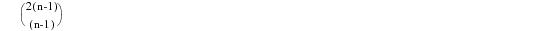More formally, it is the number of (n-1) subsets of a set of 2(n-1)elements.

To solve for it, you must substitute the following term: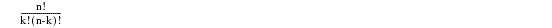where:

 This variable … Represents this term … `                  n` `             2(n-1)` `                  k` `              (n-1)`

Given the same four‑relation case used for the left-deep tree case, the result is as follows: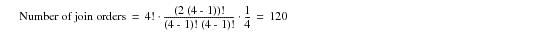Ignoring the pruning of unconstrained joins, this method produces an order of magnitude more join possibilities that can be evaluated than the left-deep tree method. Bushy trees also provide the capability of performing some joins in parallel. For example, consider the following four relation case:

`   ((A JOIN B) JOIN (C JOIN D) `

`(A JOIN B)` and `(C JOIN D)` can be dispatched for processing at the same time. A system that uses only left-deep trees cannot perform this kind of parallel join execution.

Consider the case of four relations. The number of permutations of four relations is 4! or 24. If the relations are named a, b, c, and d, then those 24 permutations are as follows:

`   abcd, abdc, acbd, acdb ... dcba`

Because the system can only join two relations at a time, these 24 permutations must be further partitioned into all their possible binary join orders as follows:

`   abcd => (((ab)c)d)`
`           ((ab)(cd))`
`           (a(b(cd)))`
`           ((a(bc))d)`
`           ((a(b(cd))`
`               .`
`               .`
`               .`

and so on.

The following graphic illustrates one possible sequence of binary joins of relations a, b, c, and d. Note the following about this description:

• The graphic is read from the bottom up.
• Intermediate join results are referred to as relations, not tables.
• The illustrated process can be described as follows:

1 Join table a with table b to create the intermediate relation ab.

2 Join table c with table d to create the intermediate relation cd.

3 Join relation ab with relation cd to create the final joined result, relation abcd.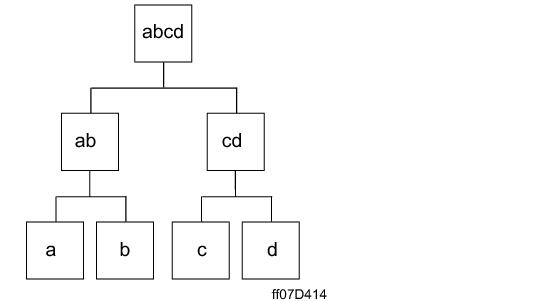Depending on table demographics and environmental costs, the Optimizer could just as likely produce the following join sequence:

1 Join table a with table b to create the intermediate relation ab.

2 Join relation ab with table c to create the intermediate relation abc.

3 Join relation abc with table d to create the final joined result, relation abcd.

The Optimizer uses various combinations of join plan search trees, sometimes mixing left-deep, bushy, and even right-deep branches within the same tree. The Optimizer is very intelligent about looking for plans and uses numerous field-proven heuristics to ensure that more costly plans are eliminated from consideration early in the costing process in order to optimize the join plan search space.

The following table illustrates the dilemma the Optimizer faces when it selects the order in which to join relations in a join plan. The table demonstrates how rapidly the possibilities for ordering binary joins escalates as the total number of relations joined increases.

To help paint a picture of how vast the number of combinations becomes as the number of tables and single‑table views increases, the following table uses the metaphor of grains of sand.

 Number of Relations Joined Number of Possible Join Orders log(Possible Join Orders) Number of Grains of Sand 3 1.2*101 1.079 12 4 1.2*102 2.079 120 10 1.8*1010 10.255 6 sand boxes 16 2.0*1020 20.301 All the sand grains of all the beaches and all the deserts in the world 64 1.2*10124 124.079 All the sand grains required to fill the known universe 128 3.966*10656 656.598 All the sand grains required to fill several parallel universes

The following graph shows the log-linear plot of the first 5 rows of data in this table: the expansion in the number of possible join orders as a function of the number of relations being joined.

Because the plot is essentially a straight line, the growth in the number of possible join orders can be characterized as exponential.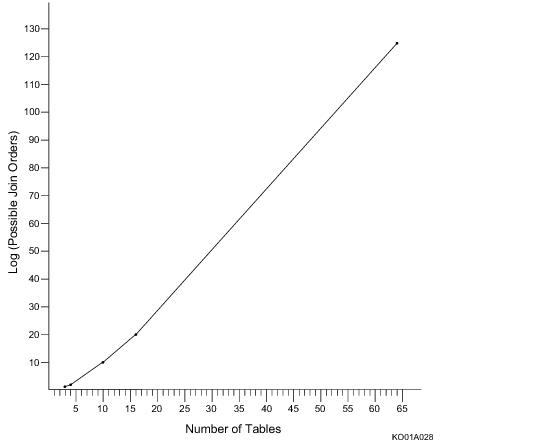The following example illustrates one way the number of participant rows in a join can be reduced.

In this example, the parts table has 4,000,000 rows. Note that there can be up to 3,990,000 rows per value (probably due to a large number of nulls in the shipnum column).

 Key Abbreviation Meaning PK Primary key FK Foreign key UPI Unique primary index SA System‑assigned

The projected columns of all 4,000,000 rows of the parts table participate in the join if the following query is submitted.

`   SELECT ...`
`   FROM shipment, part`
`   WHERE shipment.ship_num = part.ship_num;`

However, only the projected columns of 10 000 rows participate in the join if the query is modified to eliminate the nulls.

`   SELECT ...`
`   FROM shipment, part`
`   WHERE part.ship_num IS NOT NULL`
`   AND shipment.ship_num = part.ship_num;`